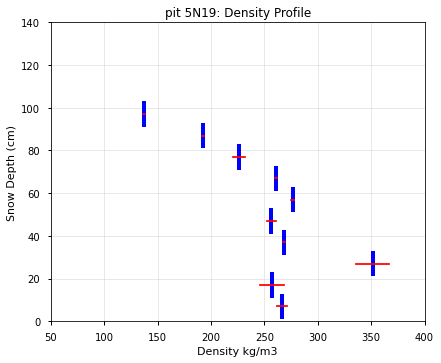# Depths and Snow Pit Data Package Contents¶

(12 minutes)

Learning Objectives:

• Tools to access data.

• Code snippets to extract and prep data.

```# standard imports
import os
from pathlib import Path
import glob
import pandas as pd
import numpy as np

#plotting imports
import matplotlib as mpl
import matplotlib.pyplot as plt
plt.style.use(['seaborn-notebook'])
```

## Access tutorial data from Zenodo¶

We’ve archived datasets used for 2021 Hackweek tutorials on Zenodo, to ensure that these tutorials can be run in the future. The following code pulls data from the Zenodo ‘record’ and unzips it:

```%%bash

# Retrieve a copy of data files used in this tutorial from Zenodo.org:

mkdir -p /tmp/tutorial-data
cd /tmp/tutorial-data
wget -q -nc -O data.zip https://zenodo.org/record/5504396/files/core-datasets.zip
unzip -q -n data.zip
rm data.zip
```
```TUTORIAL_DATA = '/tmp/tutorial-data/core-datasets'
```

From the SnowEx20 Depth Probe Landing Page, you can download data and access the User’s Guide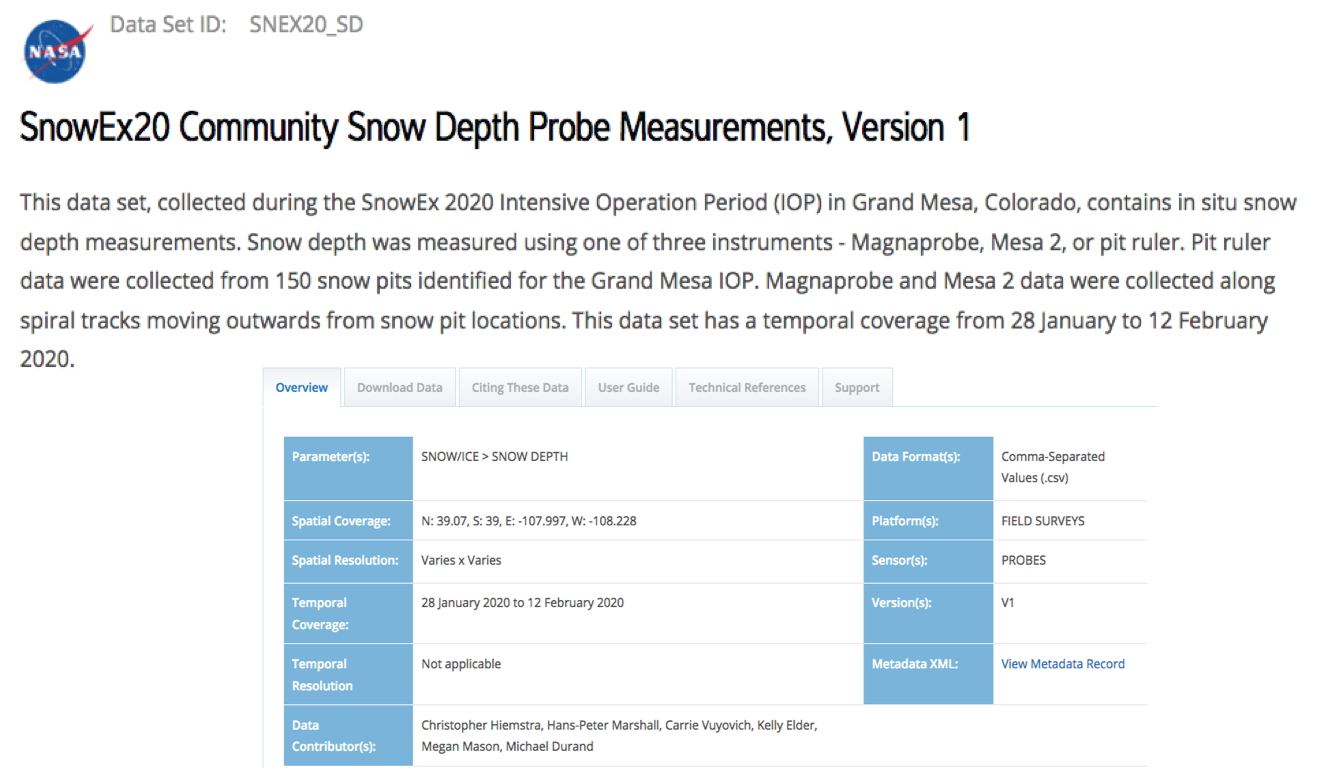The Community Snow Depth Probe data package is a single CSV with over 36,000 geolocated snow depths! Three different instrument types were used to measure depths and are recorded in the Measurement Tool column.

```#os.chmod('/home/jovyan/.netrc', 0o600) #only necessary on snowex hackweek jupyterhub
```
```#%run './scripts/nsidc-download_SNEX20_SD.001.py'
```
```#path = Path('./data/depths/')

#for filename in path.glob('*.csv'):
#    print(filename.name)
```

```df = pd.read_csv(f'{TUTORIAL_DATA}/depths/SnowEx2020_SnowDepths_COGM_alldepths_v01.csv', sep=',', header=0, parse_dates=[[2,3]]) #parse the date and time columns such that they are read in as datetime dtypes

```
```file has been read, and is ready to use.
```
```# check data types for each column
df.dtypes
```
```Date (yyyymmdd)_Time (hh:mm, local, MST)                           datetime64[ns]
Measurement Tool (MP = Magnaprobe; M2 = Mesa 2; PR = Pit Ruler)            object
ID                                                                          int64
PitID                                                                      object
Longitude                                                                 float64
Latitude                                                                  float64
Easting                                                                   float64
Northing                                                                  float64
Depth (cm)                                                                  int64
elevation (m)                                                             float64
equipment                                                                  object
Version Number                                                              int64
dtype: object
```

### Prep for Data Analysis¶

```# rename some columns for ease further down
df.rename(columns = {
'Measurement Tool (MP = Magnaprobe; M2 = Mesa 2; PR = Pit Ruler)':'Measurement Tool',
'Date (yyyymmdd)_Time (hh:mm, local, MST)': "Datetime"},
inplace = True)

# set up filter for IOP date range
start = pd.to_datetime('1/28/2020') #first day of GM IOP campaign
end   = pd.to_datetime('2/12/2020') #last day of GM IOP campaign

# filter the IOP date range
df = df[(df['Datetime'] >= start) & (df['Datetime'] <= end)]

print('DataFrame shape is: ', df.shape)
```
```DataFrame shape is:  (36388, 12)
```
Datetime Measurement Tool ID PitID Longitude Latitude Easting Northing Depth (cm) elevation (m) equipment Version Number
0 2020-01-28 11:48:00 MP 100000 8N58 -108.13515 39.03045 747987.62 4324061.71 94 3148.2 CRREL_B 1
1 2020-01-28 11:48:00 MP 100001 8N58 -108.13516 39.03045 747986.75 4324061.68 74 3148.3 CRREL_B 1
2 2020-01-28 11:48:00 MP 100002 8N58 -108.13517 39.03045 747985.89 4324061.65 90 3148.2 CRREL_B 1
3 2020-01-28 11:48:00 MP 100003 8N58 -108.13519 39.03044 747984.19 4324060.49 87 3148.6 CRREL_B 1
4 2020-01-28 11:48:00 MP 100004 8N58 -108.13519 39.03042 747984.26 4324058.27 90 3150.1 CRREL_B 1

#### Use .groupby() to sort the data set¶

```# group data by the measurement tool
gb = df.groupby('Measurement Tool', as_index=False).mean().round(1)

# show mean snow depth from each tool
gb[['Measurement Tool', 'Depth (cm)']]
```
Measurement Tool Depth (cm)
0 M2 97.0
1 MP 94.8
2 PR 94.6

```# group data by the snow pit ID

# show mean snow depth around each snow pit

#(hint: what is the pit id column called? If you're not sure you can use df.columns to see a list of column names. Consider using .head() to display only the first 5 returns)
```

#### Find depths associated with a certain measurement tool¶

```print('List of Measurement Tools: ', df['Measurement Tool'].unique())
```
```List of Measurement Tools:  ['MP' 'M2' 'PR']
```
```r = df.loc[df['Measurement Tool'] == 'PR']
print('DataFrame shape is: ', r.shape)
```
```DataFrame shape is:  (148, 12)
```
Datetime Measurement Tool ID PitID Longitude Latitude Easting Northing Depth (cm) elevation (m) equipment Version Number
37755 2020-01-30 11:24:00 PR 300001 7C15 -108.19593 39.04563 742673.94 4325582.37 100 3048.699951 ruler 1
37756 2020-01-29 15:00:00 PR 300002 6C37 -108.14791 39.00760 746962.00 4321491.00 117 3087.709961 ruler 1
37757 2020-02-09 12:30:00 PR 300003 8C31 -108.16401 39.02144 745520.00 4322983.00 98 3099.639893 ruler 1
37758 2020-01-28 09:13:00 PR 300004 6N18 -108.19103 39.03404 743137.23 4324309.43 92 3055.590088 ruler 1
37760 2020-02-10 10:30:00 PR 300006 8S41 -108.14962 39.01659 746783.00 4322484.00 95 3113.870117 ruler 1

```# find all depths recorded by the Mesa2

# (hint: copy/paste is your friend)
```

Let’s make sure we all have the same pd.DataFrame() again

```# pit ruler snow depths from Grand Mesa IOP
r = df.loc[df['Measurement Tool'] == 'PR']
print( 'DataFrame is back to only pit ruler depths')
```
```DataFrame is back to only pit ruler depths
```

### Plotting¶

```# plot pit ruler depths
ax = r.plot(x='Easting', y='Northing', c='Depth (cm)', kind='scatter', alpha=0.7, colorbar=True, colormap='PuBu', legend=True)
ax.set_title('Grand Mesa Pit Ruler Depths')
ax.set_xlabel('Easting [m]')
ax.set_ylabel('Northing [m]')
plt.show()

print('Notice the point on the far right - that is the "GML" or Grand Mesa Lodge pit where all instruments were deployed for a comparison study. pitID=GML')
```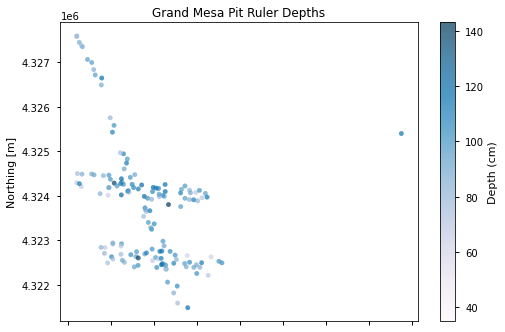```Notice the point on the far right - that is the "GML" or Grand Mesa Lodge pit where all instruments were deployed for a comparison study. pitID=GML
```
```# plot histogram of pit ruler depths
ax = r['Depth (cm)'].plot.hist(bins=25)
ax.grid()
ax.set_title('Grand Mesa Pit Ruler Depths')
ax.set_xlabel('Snow Depth (cm)')
ax.set_ylabel('Frequency')
```
```Text(0, 0.5, 'Frequency')
```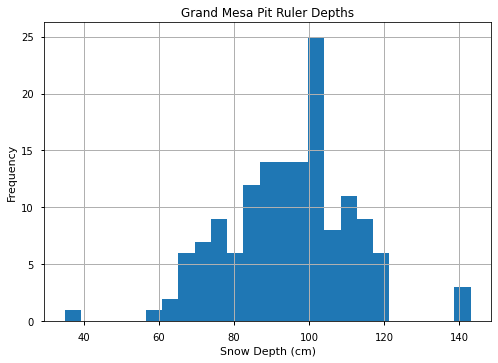From the SnowEx20 Snow Pit Landing Page, you can download data and access the User’s Guide.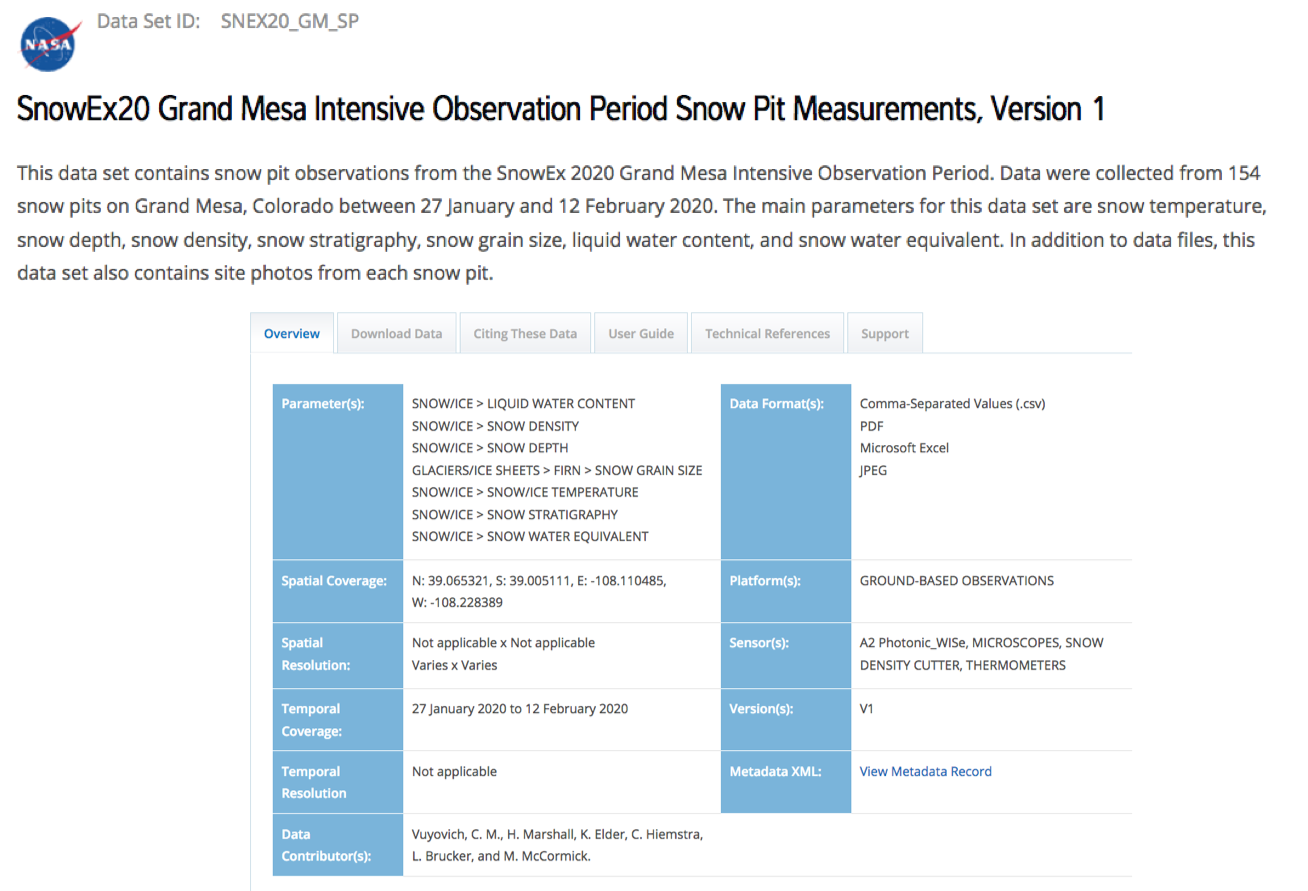```# load snow pit data
```

### Don’t want to work with all the files? Method to filter files¶

```# what files would you like to find?
parameter = 'temperature'
pitID = '5N19'
date = '20200128'

path = '{}/pits/SnowEx20_SnowPits_GMIOP_{}_{}_{}_v01.csv'.format(TUTORIAL_DATA, date, pitID, parameter)
```

### Read the Pit Parameter File¶

```t = pd.read_csv(path, header=7)
t
```
# Height (cm) Temperature (deg C)
0 102 -9.5
1 100 -9.4
2 90 -11.5
3 80 -7.5
4 70 -5.2
5 60 -4.1
6 50 -3.5
7 40 -3.0
8 30 -2.5
9 20 -2.1
10 10 -1.5
11 0 -0.8

### Plotting¶

```# plot temperature
ax = t.plot(x='Temperature (deg C)',y='# Height (cm)', legend=False)
ax.set_aspect(0.4)
ax.grid()
ax.set_title('pit {}: Temperature Profile'.format(pitID))
ax.set_xlabel('Temperature (deg C)')
ax.set_ylabel('Snow Depth (cm)')
```
```Text(0, 0.5, 'Snow Depth (cm)')
```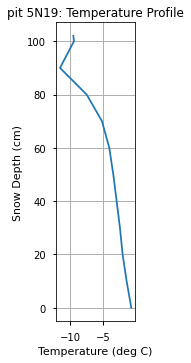```# grab a different pit parameter file
parameter = 'density'
path = '/tmp/tutorial-data/core-datasets/pits/SnowEx20_SnowPits_GMIOP_{}_{}_{}_v01.csv'.format(date, pitID, parameter)
d
```
# Top (cm) Bottom (cm) Density A (kg/m3) Density B (kg/m3) Density C (kg/m3)
0 102.0 92.0 136.0 138.0 NaN
1 92.0 82.0 193.0 192.0 NaN
2 82.0 72.0 232.0 221.0 NaN
3 72.0 62.0 262.0 260.0 NaN
4 62.0 52.0 275.0 278.0 NaN
5 52.0 42.0 261.0 252.0 NaN
6 42.0 32.0 267.0 269.0 NaN
7 32.0 22.0 336.0 367.0 NaN
8 22.0 12.0 268.0 246.0 NaN
9 12.0 2.0 262.0 271.0 NaN
```# get the average density
d['Avg Density (kg/m3)'] = d[['Density A (kg/m3)', 'Density B (kg/m3)', 'Density C (kg/m3)']].mean(axis=1, skipna=True)
d
```
# Top (cm) Bottom (cm) Density A (kg/m3) Density B (kg/m3) Density C (kg/m3) Avg Density (kg/m3)
0 102.0 92.0 136.0 138.0 NaN 137.0
1 92.0 82.0 193.0 192.0 NaN 192.5
2 82.0 72.0 232.0 221.0 NaN 226.5
3 72.0 62.0 262.0 260.0 NaN 261.0
4 62.0 52.0 275.0 278.0 NaN 276.5
5 52.0 42.0 261.0 252.0 NaN 256.5
6 42.0 32.0 267.0 269.0 NaN 268.0
7 32.0 22.0 336.0 367.0 NaN 351.5
8 22.0 12.0 268.0 246.0 NaN 257.0
9 12.0 2.0 262.0 271.0 NaN 266.5
```def plot_density(ax, dataframe):

'''
This function helps you plot density profiles from snow pits. Use it to iterate through
DataFrame rows and plot the density for each top and bottom segment.

'''

for index, row in dataframe.iterrows():
# plot blue bars to represent 10cm density intervals
top = row['# Top (cm)']
bottom = row['Bottom (cm)']
dens = row["Avg Density (kg/m3)"]
ax.plot([dens, dens],[bottom, top], color='blue', linewidth=4)

# plot a red cross to show the spread between A and B samples
densA = row["Density A (kg/m3)"]
densB = row["Density B (kg/m3)"]
middle = bottom + 5.
ax.plot([densA, densB],[middle,middle], color='red')

return ax

fig, ax = plt.subplots()
ax = plot_density(ax, d)
ax.set_xlim(50, 400)
ax.set_ylim(0, 140)
ax.grid(color='lightgray', alpha=.5)
ax.set_aspect(2)
ax.set_title('pit {}: Density Profile'.format(pitID))
ax.set_xlabel('Density kg/m3')
ax.set_ylabel('Snow Depth (cm)')
```
```Text(0, 0.5, 'Snow Depth (cm)')
```